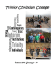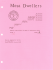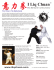# Sample Midterm CSE 680: Introduction to Algorithms and Data Structures

## Transcription

Sample Midterm CSE 680: Introduction to Algorithms and Data Structures
```Sample Midterm
CSE 680: Introduction to Algorithms and Data Structures
1.
Use a recursion tree to determine an asymptotically tight bound to the recurrence
T(n) = T(n/3) + T(2n/3) + O(n). Use the substitution method to verify your answer.
O(n)
T(n)
O(n/3)+O(2n/3)=O(n)
O(n)
T(n/3)
T(n/9)
T(2n/3)
T(2n/9)
T(2n/9)
T(4n/9)
T(8n/27)
Height of tree is log2/3(n), so total is O(n log2/3(n)).
2.
Solve the following recurrences by giving tight Θ-notation bounds, using the Master method if possible.
(a) T(n) = T(n/3) + T(2n/3) + 2n
T(n) < 2T(2n/3) + 2n => Case 1, but could also state that the Master method does not apply.
(b) T (n)=3T (n/5)+lg2n
log5(3) = 0.68 => Case 1 since lg2n < n0.68 for n > 29. T(n) = ( n0.68).
(c) T (n)=7T (n/2)+n3
lg(7) = 2.8 => Case 3. Check that 7(n/2)3 < n3. Yes, so T(n) = (n3).
3.
(a)
(b)
(c)
(d)
(e)
(f)
(g)
(h)
4.
Rank the following functions by order of growth; that is find an arrangement g1, g2, …, g8 of the functions
satisfying g1 = Ω(g2), g2 = Ω(g3), …, g7 = Ω(g8). Partition your list into equivalence classes such that gi(n) and gj(n)
are in the same class if and only if gi(n) = Θ(gj(n)).
n/100
n2
n lg n
2
2n
3log3 n
n2 − 9000n
210n
 (e) Note, this is g1.
 (b) and (g)
 (c)
 (a), (f) and (h)
 (d)
What is the time complexity (as a function of n) for the following algorithm? Please give a tight bound. Be sure
MysteryAlg(n)
x = 0
for i = 1 to n
for j = 1 to i
x = x * 2
end for
k = 1
while k <= n^2
x = x - 1
k = k + 1
end while
end for
return x
T(n) = 1 + n(n+1)/2 + n + 2n3 = (n3)
5.
Write a recursion formula for the running time T(n) of the function NoNeed2, whose code is below. Prove using
the iterative method that T(n) =  (n3).
NoNeed2(int n) {
if (n < 3) return;
for(i = 1; i < n; i++)
for( j = 1; j < n; j++)
print(“*” );
NoNeed2(n-3);
}
T(n) = T(n-3) + (n2)
Guess T(n) = cn3.
T(n) = n2 + c(n-3)3 = n2 + c(n3 + 6n2 – 18n – 27) > cn3 – need an adjustment!
Guess T(n) = cn3 – bn2. Which will work as long as b > 6c+1.
6.
What is the Big-O of the following code?
int total = 0;
for(int i = 0; i < N; i++)
for(int j = 0; j < 5; j++)
for(int k = 0; k < N; k++)
for(int m = 1; m <= N; m = m * 2)
total++;
T(n) = 5n2lg(n)
7.
Fill in the following table. Each box should have a “yes” or “no” indicating whether or not the condition
at the top of the row is true.
A
B
A=O(B)
A=(B)
A=(B)
n
1
No
Yes
No
n2
n lgn
No
Yes
No
n+n2
n3
Yes
No
No
n
200n + n/2
Yes
Yes
Yes
n.5
lg n
No
Yes
No
2n
Yes
No
No
10
n10
8.
Show Merge sort on the following array. Carefully explain each step.
3, 4, 8, 1, 6, 7, 2, 5
3,4,8,1
6,7,2,5
3,4 1,8 6,7 2,5
1,3,4,8 2,5,6,7
1,2,3,4,5,6,7,8
9.
Show a decision tree for finding the minimum of three numbers a, b, and c.
a<b
a<c
a
10.
b<c
c
b
c
Given the structure of a heap as sketched below, where the second-smallest value in the set is marked
with a 2. Mark a 4 for each node that can possibly contain the fourth-smallest value in the set.
Assume that there are no duplicate node values.
2
The shaded nodes are the answer. Any of the nodes shown below the 2 could be the 4th smallest
element. If the left child of the root is the 3rd smallest element, then either of its children, but not its
grandchildren, could be the 4th smallest. If the 3rd smallest is under the 2, then the left child of the root
could also be the 4th smallest.
```

### ICSE Computer Application Sample Papers SECTION A (40 Marks)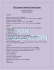### IE 201 – Sample Midterm Questions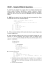### Lecture-10### Wizard 5 Soldier James Krutzworth Steelbelly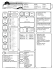### How to build, deploy and test SCOS 2000 www.visionspace.com April 30, 2014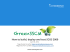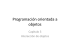### CSC 171 Sample Quiz 6 C Language Programming for Engineers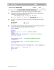### -: Multiple Choice Questions:- Computer Science (C++) First Unit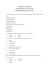### Section Solutions 6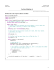### Field Service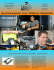### Lab 10 - ocal.info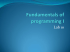### Computer Science - Class - XII Sample Paper - 3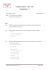### Note to Little Miss Hot Pants### Picture Lab - Student Answer Form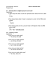### CS 1428 Credit By Exam: Sample Questions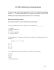### sample final exam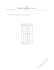### 15-122 : Principles of Imperative Computation, Spring 2013 Homework 2 Theory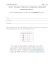### File Systems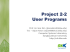### Computer Science - Class - XII Sample Paper - 1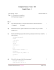### Trinity Christian College Interim 2015: January 6 - 19